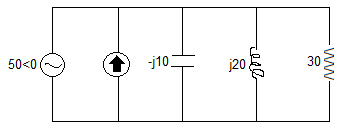# Thevenin equivalent

For the circuit below, can someone please help me understand why Zeq = 0 (in words...not by solving):I know Vth = 50<0° since this is the voltage drop across the parallel elements. I don't understand why the equivalent impedance would be 0. I know when you shut off a voltage source, elements in parallel with it also go away. does this have anything to do with it?

Zryn
Gold Member
http://en.wikipedia.org/wiki/Th%C3%A9venin%27s_theorem" [Broken]

To calculate the equivalent circuit, the resistance and voltage are needed, so two equations are required. These two equations are usually obtained by using the following steps, but any conditions placed on the terminals of the circuit should also work:

1. Calculate the output voltage, VAB, when in open circuit condition (no load resistor—meaning infinite resistance). This is VTh.
2. Calculate the output current, IAB, when the output terminals are short circuited (load resistance is 0). RTh equals VTh divided by this IAB.

The equivalent circuit is a voltage source with voltage VTh in series with a resistance RTh.

Step 2 could also be thought of as:

2a. Replace voltage sources with short circuits, and current sources with open circuits.
2b. Calculate the resistance between terminals A and B. This is RTh.

So you know the Thevenin Voltage already. In step two you can short all voltage sources, open all current sources (as per step 2a.) and then comment on the resistance of the remaining circuit.

Last edited by a moderator:
NascentOxygen
Staff Emeritus
What is the element represented by a circle with an arrow in it?

NascentOxygen
Staff Emeritus
What is the element represented by a circle with an arrow in it?

What is the element represented by a circle with an arrow in it?

it's a current source

http://en.wikipedia.org/wiki/Th%C3%A9venin%27s_theorem" [Broken]

So you know the Thevenin Voltage already. In step two you can short all voltage sources, open all current sources (as per step 2a.) and then comment on the resistance of the remaining circuit.

so is it because when you short the voltage source the elements in parallel are shut off, too?

Last edited by a moderator:
Zryn
Gold Member
It's only a matter of calculating the parallel resistance as per normal:

1/R(t) = 1 / R(short) + 1 / R(open) + 1 / R(C) + 1 / R(L) + 1 / R(R)

R(t) = 1 / [1 / 0 + 1 / inf + 1 / -j10 + 1 / j20 + 1 / 30]

R(t) = 1 / [(1 + 0 + 0 + 0 + 0)/0] (put all 5 fractions over a common denominator)

R(t) = 1 / [1 / 0]

R(t) = 1 * [0 / 1]

R(t) = 0

You could have just 'seen' that by knowing that the short circuit from the voltage source will bypass all the other elements in the circuit, since current will follow the path of least resistance and split down parallel paths in inverse proportionality to the magnitude of the resistance of each path. I guess 'shut off' is correct, but you could word it better.

NascentOxygen
Staff Emeritus
(Please don't say it doesn't matter. I can see that! I'd still like to know the question before I state the answer.)